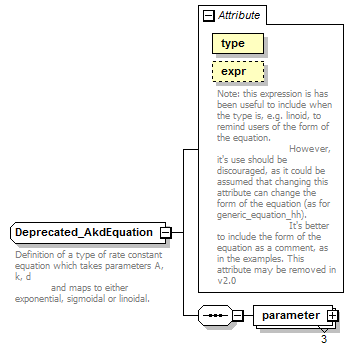# Deprecated_AkdEquation

 ```Definition of a type of rate constant equation which takes parameters A, k, d and maps to either exponential, sigmoidal or linoidal.```

## Model

Children: parameter## Attributes

QName Type Fixed Default Use Inheritable Annotation
expr xs:string optional
 ```Note: this expression is has been useful to include when the type is, e.g. linoid, to remind users of the form of the equation. However, it's use should be discouraged, as it could be assumed that changing this attribute can change the form of the equation (as for generic_equation_hh). It's better to include the form of the equation as a comment, as in the examples. This attribute may be removed in v2.0```
type Deprecated_CoreEquationType required

## Used By

 Element Deprecated_RateConstantEqnChoice/parameterised_hh

## Source

 ``` Definition of a type of rate constant equation which takes parameters A, k, d and maps to either exponential, sigmoidal or linoidal. Note: this expression is has been useful to include when the type is, e.g. linoid, to remind users of the form of the equation. However, it's use should be discouraged, as it could be assumed that changing this attribute can change the form of the equation (as for generic_equation_hh). It's better to include the form of the equation as a comment, as in the examples. This attribute may be removed in v2.0 ```